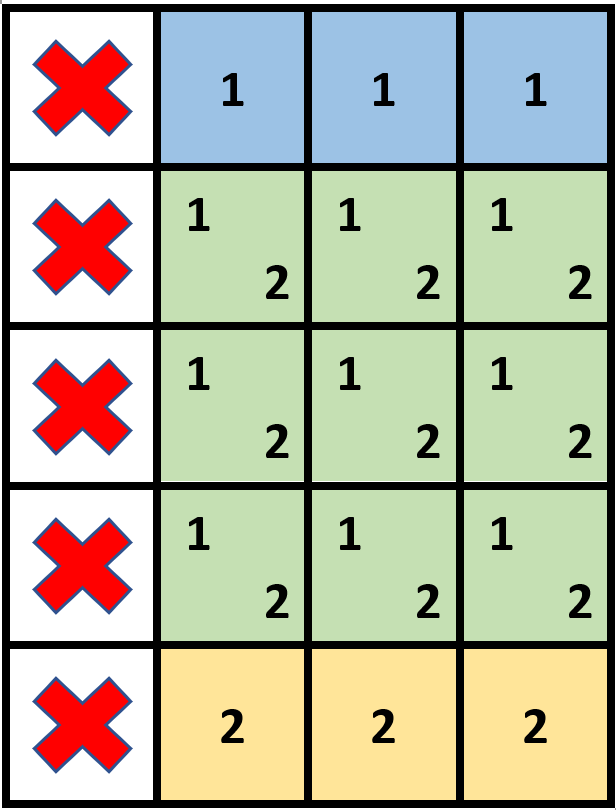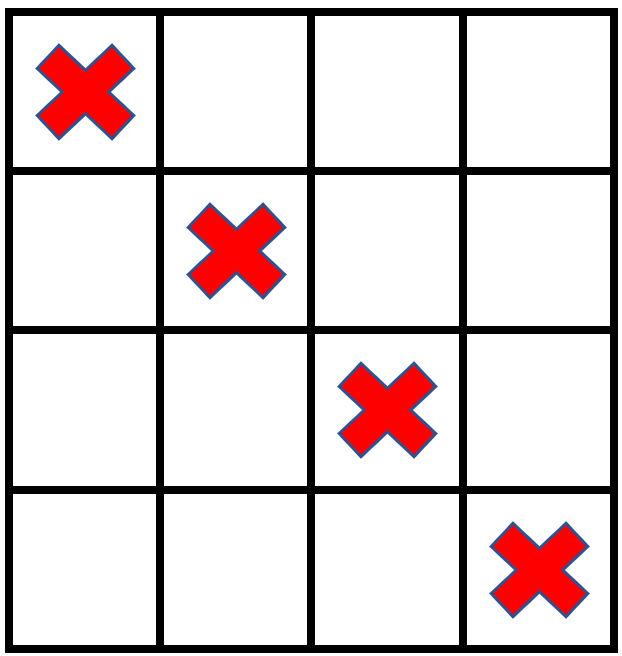##### Welcome to Subscribe On Youtube

Formatted question description: https://leetcode.ca/all/2132.html

# 2132. Stamping the Grid

• Difficulty: Hard.
• Related Topics: Array, Greedy, Matrix, Prefix Sum.
• Similar Questions: Maximal Square, Bomb Enemy, Matrix Block Sum.

## Problem

You are given an m x n binary matrix grid where each cell is either 0 (empty) or 1 (occupied).

You are then given stamps of size stampHeight x stampWidth. We want to fit the stamps such that they follow the given restrictions and requirements:

• Cover all the empty cells.

• Do not cover any of the occupied cells.

• We can put as many stamps as we want.

• Stamps can overlap with each other.

• Stamps are not allowed to be rotated.

• Stamps must stay completely inside the grid.

Return true if it is possible to fit the stamps while following the given restrictions and requirements. Otherwise, return false.

Example 1:Input: grid = [[1,0,0,0],[1,0,0,0],[1,0,0,0],[1,0,0,0],[1,0,0,0]], stampHeight = 4, stampWidth = 3
Output: true
Explanation: We have two overlapping stamps (labeled 1 and 2 in the image) that are able to cover all the empty cells.


Example 2:Input: grid = [[1,0,0,0],[0,1,0,0],[0,0,1,0],[0,0,0,1]], stampHeight = 2, stampWidth = 2
Output: false
Explanation: There is no way to fit the stamps onto all the empty cells without the stamps going outside the grid.


Constraints:

• m == grid.length

• n == grid[r].length

• 1 <= m, n <= 105

• 1 <= m * n <= 2 * 105

• grid[r][c] is either 0 or 1.

• 1 <= stampHeight, stampWidth <= 105

## Solution

• class Solution {
private boolean canPaved(int[][] grid, int is, int js, int ie, int je) {
for (int i = is; i <= ie; i++) {
for (int j = js; j <= je; j++) {
if (grid[i][j] == 1) {
return true;
}
}
}
return false;
}

public boolean possibleToStamp(int[][] grid, int h, int w) {
int rl = grid.length;
for (int i = 0; i < grid.length; i++) {
int[] row = grid[i];
int prev = -1;
for (int j = 0; j < row.length; j++) {
if (row[j] == 0) {
if (j + 1 < rl
&& row[j + 1] == 1
&& j - w + 1 >= 0
&& i + 1 < grid.length
&& grid[i + 1][j] == 1
&& i - h + 1 >= 0
&& canPaved(grid, i - h + 1, j - w + 1, i, j)) {
return false;
}
continue;
}
if (1 < j - prev && j - prev <= w) {
return false;
}
prev = j;
}
if (1 < row.length - prev && row.length - prev <= w) {
return false;
}
}
for (int i = 0; i < rl; i++) {
int prev = -1;
for (int j = 0; j < grid.length; j++) {
if (grid[j][i] == 0) {
continue;
}
if (1 < j - prev && j - prev <= h) {
return false;
}
prev = j;
}
if (1 < grid.length - prev && grid.length - prev <= h) {
return false;
}
}
return true;
}
}

• Todo

• class Solution:
def possibleToStamp(
self, grid: List[List[int]], stampHeight: int, stampWidth: int
) -> bool:
m, n = len(grid), len(grid)
s = [ * (n + 1) for _ in range(m + 1)]
for i, row in enumerate(grid):
for j, v in enumerate(row):
s[i + 1][j + 1] = s[i + 1][j] + s[i][j + 1] - s[i][j] + v

d = [ * (n + 1) for _ in range(m + 1)]
for i, row in enumerate(grid):
for j, v in enumerate(row):
if v == 0:
x, y = i + stampHeight, j + stampWidth
if x <= m and y <= n and s[x][y] - s[x][j] - s[i][y] + s[i][j] == 0:
d[i][j] += 1
d[i][y] -= 1
d[x][j] -= 1
d[x][y] += 1

cnt = [ * (n + 1) for _ in range(m + 1)]
for i, row in enumerate(grid):
for j, v in enumerate(row):
cnt[i + 1][j + 1] = cnt[i + 1][j] + cnt[i][j + 1] - cnt[i][j] + d[i][j]
if v == 0 and cnt[i + 1][j + 1] == 0:
return False
return True

############

# 2132. Stamping the Grid
# https://leetcode.com/problems/stamping-the-grid/

class Solution:
def possibleToStamp(self, grid: List[List[int]], H: int, W: int) -> bool:
rows, cols = len(grid), len(grid)
prefix = [ * (cols + 1) for _ in range(rows + 1)]

for x in range(rows):
for y in range(cols):
prefix[x + 1][y + 1] = prefix[x + 1][y] + prefix[x][y + 1] - prefix[x][y] + grid[x][y]

diff = [ * (cols + 1) for _ in range(rows + 1)]

for x in range(rows - H + 1):
for y in range(cols - W + 1):
rect = prefix[x + H][y + W] - prefix[x + H][y] - prefix[x][y + W] + prefix[x][y]

if rect == 0:
diff[x][y] += 1
diff[x + H][y] -= 1
diff[x][y + W] -= 1
diff[x + H][y + W] += 1

for x in range(rows + 1):
for y in range(cols):
diff[x][y + 1] += diff[x][y]

for y in range(cols + 1):
for x in range(rows):
diff[x + 1][y] += diff[x][y]

for x in range(rows):
for y in range(cols):
if grid[x][y] == 0 and diff[x][y] == 0:
return False

return True



Explain:

nope.

Complexity:

• Time complexity : O(n).
• Space complexity : O(n).